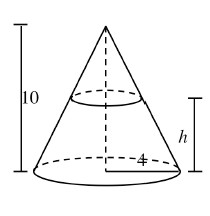### Home > CALC > Chapter 4 > Lesson 4.1.2 > Problem4-25

4-25.

A cone has a height of $10$ inches and a radius of $4$ inches. If a plane cuts the cone $h$ inches above the base of the cone, write an expression for the area of the circular cross-section.Area of a circle $= πr^2$

Sketch the radius and look for a geometric way to find its length.

Do you see the similar triangles?

Similar triangles have proportional sides, write a proportion to solve for $r$. Be careful! The height of the small triangle is not $h$.